# R R 5. To compute 1 = lim 2 COS dr and J = lim 22+1...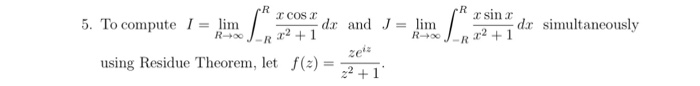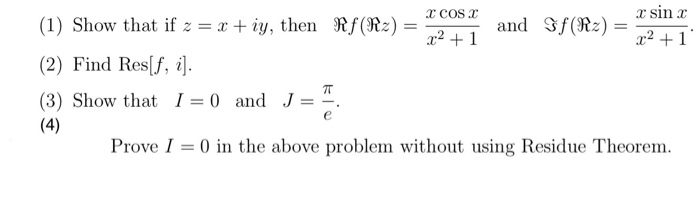R R 5. To compute 1 = lim 2 COS dr and J = lim 22+1 sinc dx simultaneously .22 +1 R R0 R R using Residue Theorem, let f(x) 22 +1
C COSC sinc (1) Show that if z = x + iy, then Rf(R2) = and Sf(R2) = x2 +1 x2 +1 (2) Find Res[f, i]. (3) Show that I = 0 and J (4) Prove I = 0) in the above problem without using Residue Theorem. IT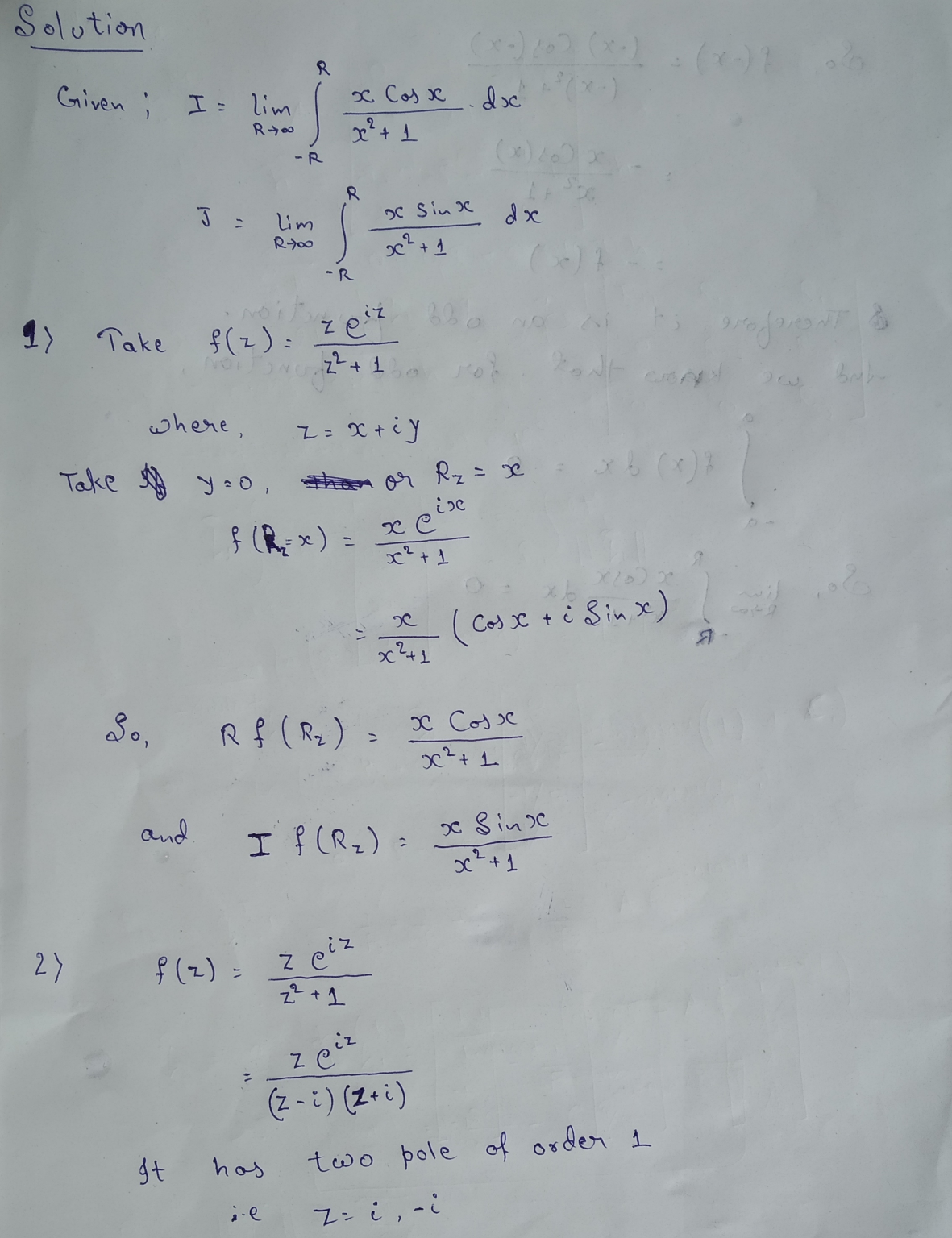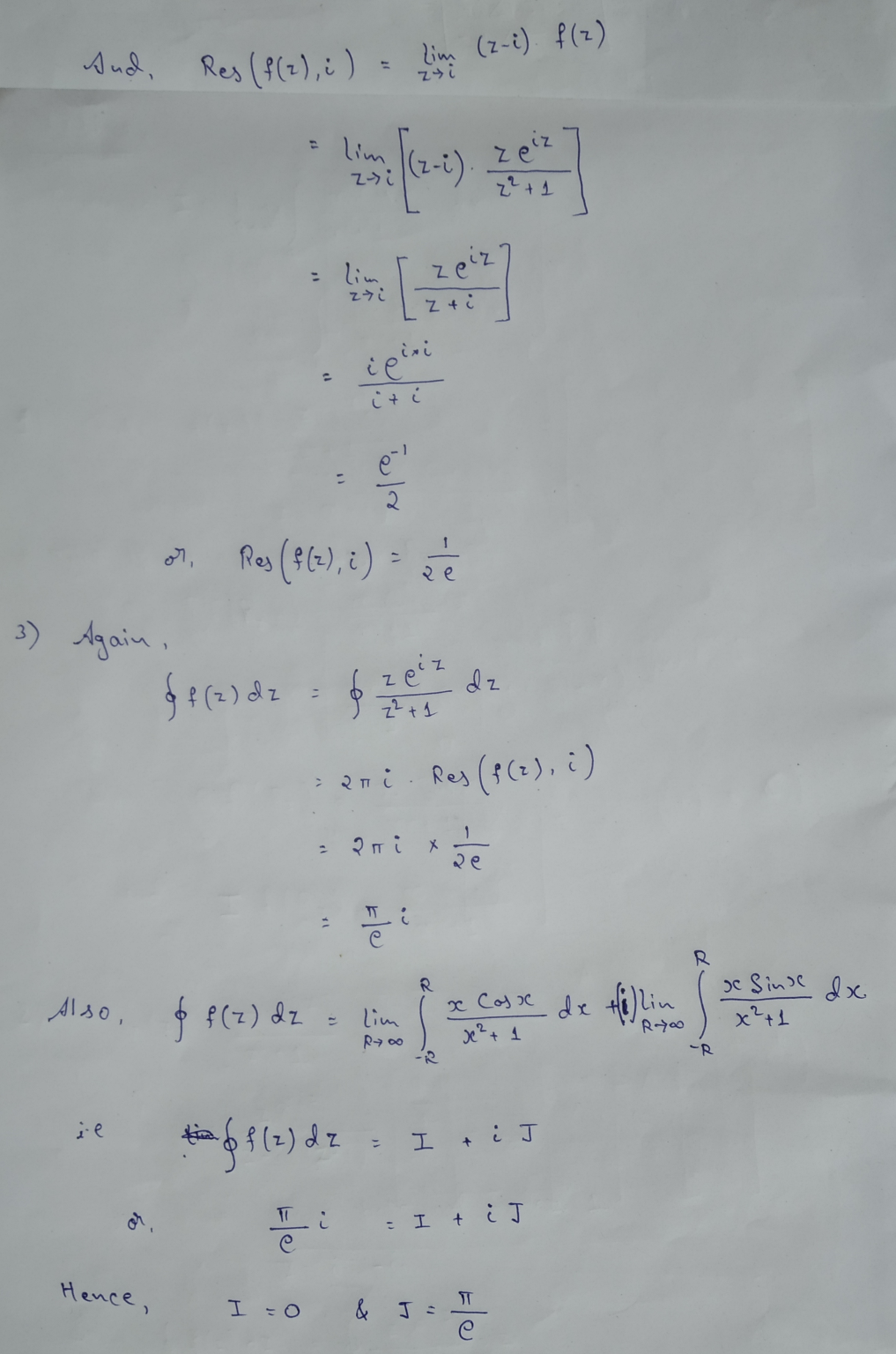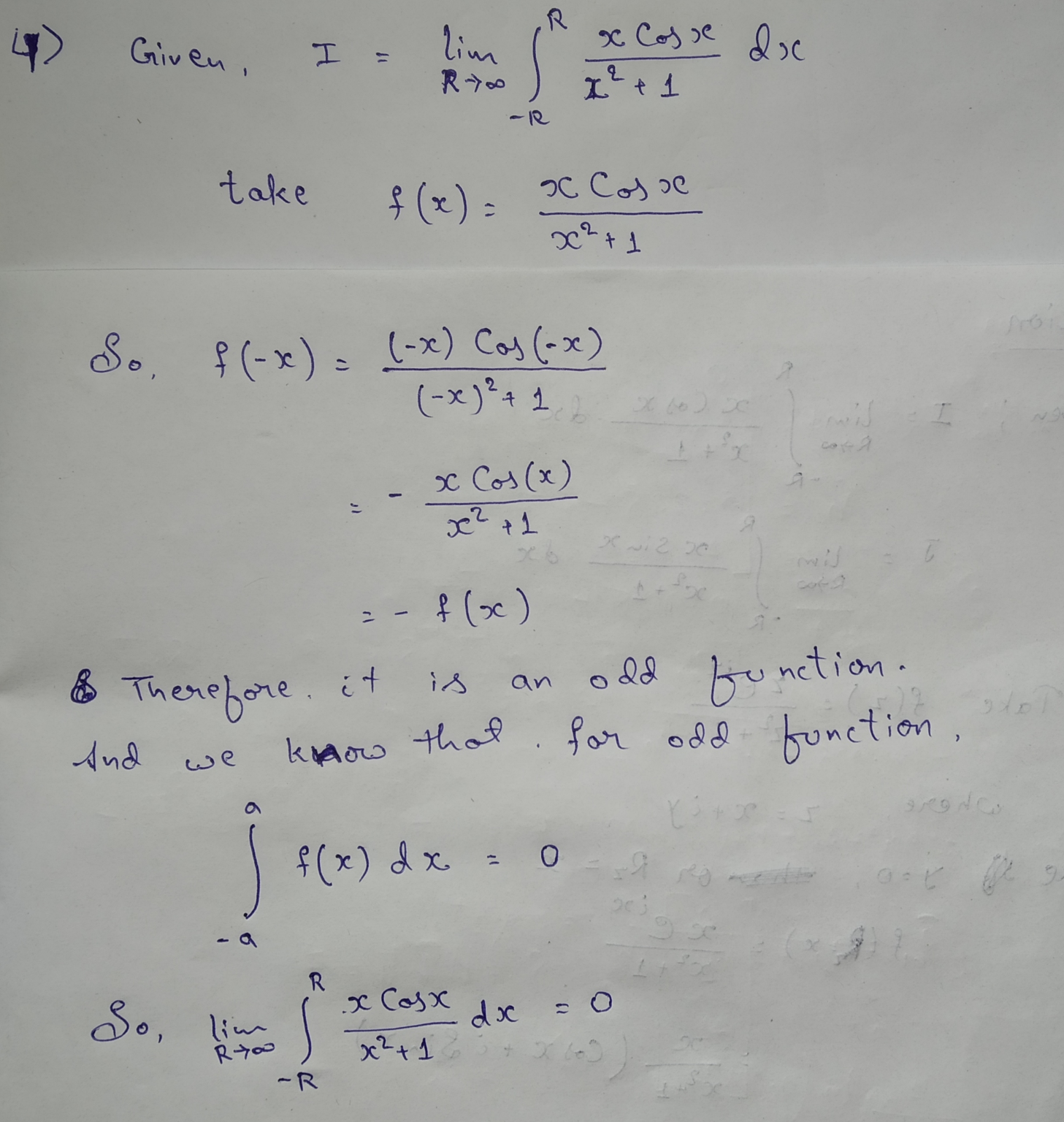##### Add Answer of: R R 5. To compute 1 = lim 2 COS dr and J = lim 22+1...
Similar Homework Help Questions
• ### Please solve this without copying the answer of exact same question in Chegg since I would...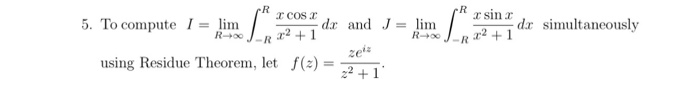Please solve this without copying the answer of exact same question in Chegg since I would like to know the other way of solution. Thank you. R R sinc dx simultaneously 2+1 R R0 R R 2 COS 5. To compute 1 = lim 22+1 dar and J = lim zez using Residue Theorem, let f() 22 +1 C COSC sinc (1) Show that if z = x + iy, then Rf(R2) = and Sf(R2) = x2 +1 x2 +1...

• ### 5. (a) Show that the following improper real integral is absolutely convergent cos 2x dr I...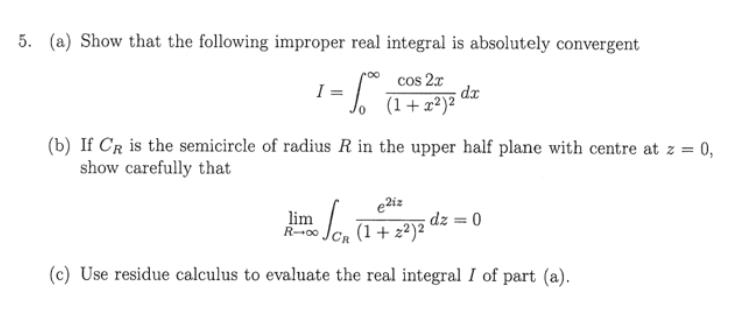5. (a) Show that the following improper real integral is absolutely convergent cos 2x dr I (1+?}% " (b) If CR is the semicircle of radius R in the upper half plane with centre at z = 0, show carefully that e2iz lim R00JCR (1+ z2)2 d% = 0 (c) Use residue calculus to evaluate the real integral I of part (a) 5. (a) Show that the following improper real integral is absolutely convergent cos 2x dr I (1+?}% "...

• ### (2 pts) Calculate the circulation, rF dr, in two ways, directly and using Stokes' Theorem. The vector field F (...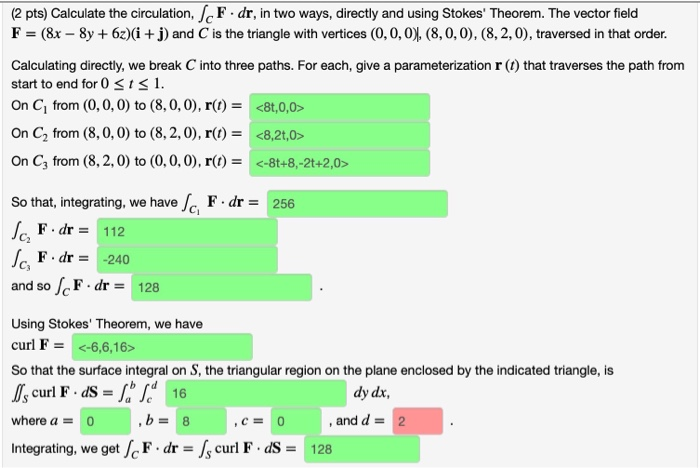(2 pts) Calculate the circulation, rF dr, in two ways, directly and using Stokes' Theorem. The vector field F (8x-8y+62)(i + j) and C is the triangle with vertices (0,0,0), (8, 0, 0), (8,2,0), traversed in that order. Calculating directly, we break C into three paths. For each, give a parameterization r (t) that traverses the path from start to end for 0sts 1 On Ci from (0,0, 0) to (8,0,0), r(t) = <8t,0,0> On C2 from (8, 0, 0)...

• ### Problem 5. [2+2 pts) Compute the following limits: 2 cos(x)-2+x2 (i) lime-0 22 sin?(x) (ii) lim:700(1...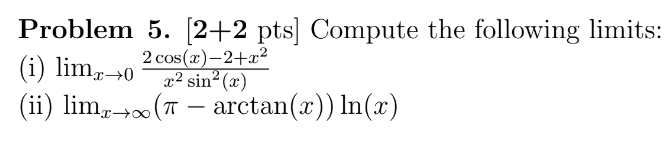Problem 5. [2+2 pts) Compute the following limits: 2 cos(x)-2+x2 (i) lime-0 22 sin?(x) (ii) lim:700(1 – arctan(x)) ln(x)

• ### 2017 is the power of (1 + x^2) Exercise 9. (i) Evaluate dr (ii) Show that the following improper integral converges roo...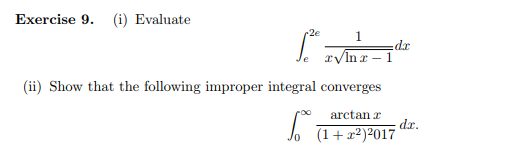2017 is the power of (1 + x^2) Exercise 9. (i) Evaluate dr (ii) Show that the following improper integral converges roo arctan r dx. Jo (1+r2)2017 Exercise 9. (i) Evaluate dr (ii) Show that the following improper integral converges roo arctan r dx. Jo (1+r2)2017

• ### F) 3w-5 w-25 (2) Which of the following definite integrals cannot be evaluated using the Fundamental Theorem of Cal...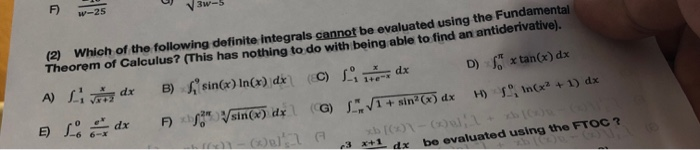F) 3w-5 w-25 (2) Which of the following definite integrals cannot be evaluated using the Fundamental Theorem of Calculus? (This has nothing to do with being able to find an antiderivative), D) S B) sin() In(x) dx (C) x tan(x) dx A) dr dx 1+e In(x2 +1) dx sin(® dx\ (G) J V+ sin H) F) 2 dx o )u xb [(x)1-(x)ul, 3 x+1dx be evaluated using the FTOC ? C) F) 3w-5 w-25 (2) Which of the following definite...

• ### 011 TA -1 -2 2. (5 points) Use the limit definition of the derivative f'(x)= lim...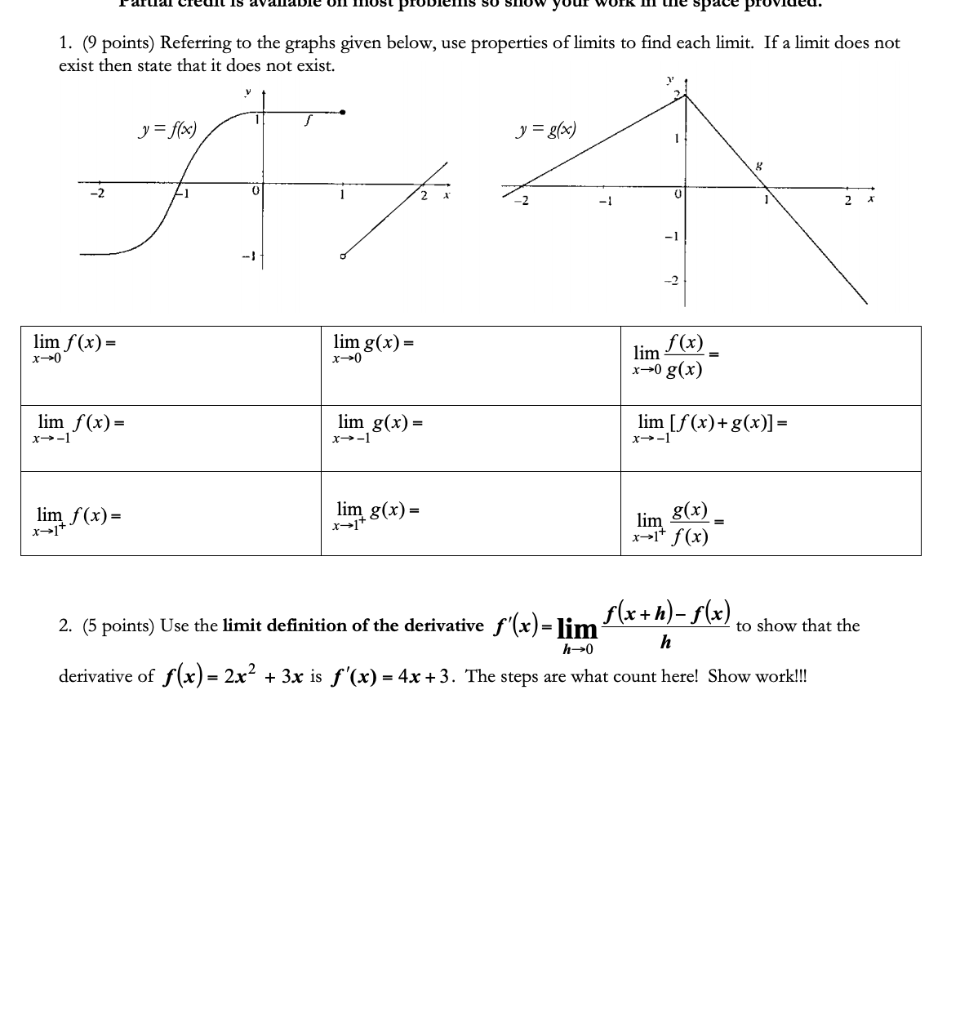011 TA -1 -2 2. (5 points) Use the limit definition of the derivative f'(x)= lim f (x+h)-f(x) to show that the 1. (9 points) Referring to the graphs given below, use properties of limits to find each limit. If a limit does not exist then state that it does not exist. y = f(x) y = g(x) lim f(x)= lim g(x) = f(x) x- lim x+0 g(x) lim lim g(x) = lim [f(x)+g(x)] = x-1 lim f(x) = lim...

• ### Let f: (0,1)--> R be an arbitrary function with the properties: Find lim(x-->0) f(x)/x.

Let f: (0,1)--> R be an arbitrary function with the properties:i)Lim(x-->0)f(x)=0ii)Lim(x-->0) 1/x(f(x)-f(x/2))=0Find lim(x-->0) f(x)/x.Hint: Use the definition of f'(a) and the Mean Value Theorem

• ### Let f: (0,1)--> R be an arbitrary function with the properties.....Find lim(x-->0) f(x)/x (Use...

Let f: (0,1)--> R be an arbitrary function with the properties:i)Lim(x-->0)f(x)=0ii)Lim(x-->0) 1/x(f(x)-f(x/2))=0Find lim(x-->0) f(x)/x.Hint: Use the definition of f'(a) and the Mean Value Theorem

• ### 1. Consider the function -F5 sin(r) for r f(x) =2 for 1< 3 2-25 for 3...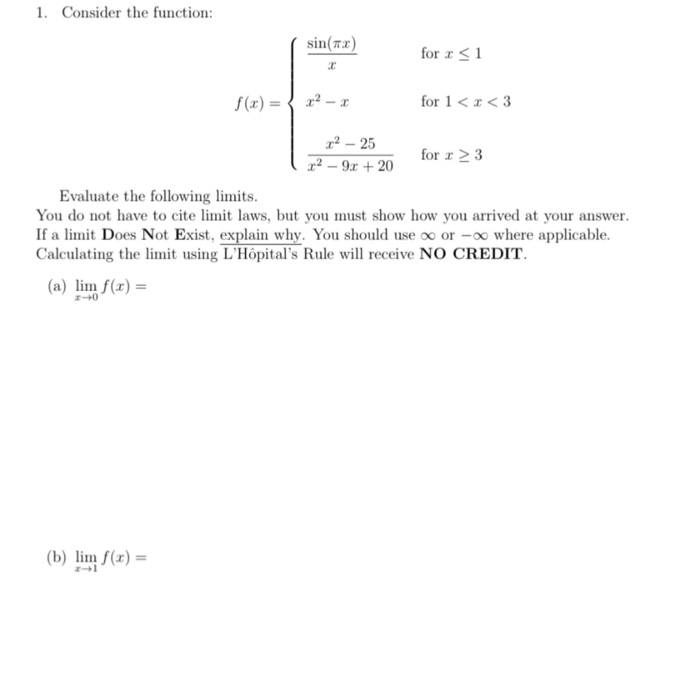1. Consider the function -F5 sin(r) for r f(x) =2 for 1< 3 2-25 for 3 x2 -9x + 20 Evaluate the following limits You do not have to cite limit laws, but you must show how you arrived at your answer If a limit Does Not Exist, explain why. You should use oo or -oo where applicable Calculating the limit using L'Hopital's Rule will receive NO CREDIT. (a) lim f(x) r-+0 (b) lim f(x)= z-1 (e) lim f(z) (d)...

Free Homework App